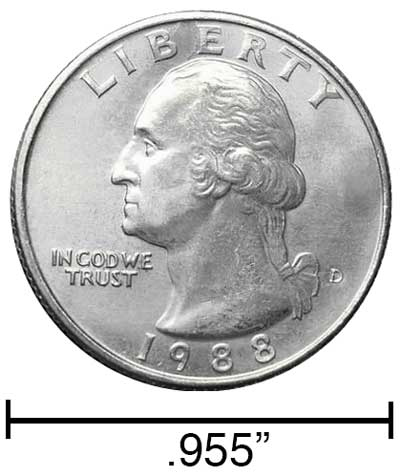# Micrometers to Inches Conversion

Enter the length in micrometers below to get the value converted to inches.

Results in Inches:1 µm = 3.937E-5 in
Hint: use a scientific notation calculator to convert E notation to decimal

## How to Convert Micrometers to InchesTo convert a micrometer measurement to an inch measurement, divide the length by the conversion ratio. One inch is equal to 25,400 micrometers, so use this simple formula to convert:

inches = micrometers ÷ 25,400

The length in inches is equal to the micrometers divided by 25,400.

For example, here's how to convert 50,000 micrometers to inches using the formula above.
50,000 µm = (50,000 ÷ 25,400) = 1.968504"

Our inch fraction calculator can add micrometers and inches together, and it also automatically converts the results to US customary, imperial, and SI metric values.

## Micrometers

One micrometer is equal to one-millionth (1/1,000,000) of a meter, which is defined as the distance light travels in a vacuum in a 1/299,792,458 second time interval.

The micrometer, or micrometre, is a multiple of the meter, which is the SI base unit for length. In the metric system, "micro" is the prefix for 10-6. A micrometer is sometimes also referred to as a micron. Micrometers can be abbreviated as µm; for example, 1 micrometer can be written as 1 µm.

To get an idea of the actual physical length of a micrometer, one human hair is 40-50 µm thick, demonstrating how small this unit of measure is.

## Inches

An inch is a unit of linear length measure equal to 1/12 of a foot or 1/36 of a yard. Because the international yard is legally defined to be equal to exactly 0.9144 meters, one inch is equal to 2.54 centimeters.

The inch is a US customary and imperial unit of length. Inches can be abbreviated as in; for example, 1 inch can be written as 1 in.

Inches can also be denoted using the symbol, otherwise known as a double-prime. Often a double-quote (") is used instead of a double-prime for convenience. A double-prime is commonly used to express 1 in as 1″.The standard ruler has 12", and is a common measuring tool for measuring inches. They are also often measured using tape measures, which commonly come in lengths from 6' - 35'. Other types of measuring devices include scales, calipers, measuring wheels, micrometers, yardsticks, and even lasers.

We recommend using a ruler or tape measure for measuring length, which can be found at a local retailer or home center. Rulers are available in imperial, metric, or combination with both values, so make sure you get the correct type for your needs.

Need a ruler? Try our free downloadable and printable rulers, which include both imperial and metric measurements.

## Micrometer to Inch Conversion Table

Micrometer measurements converted to inches
Micrometers Inches
1 µm 0.00003937"
2 µm 0.00007874"
3 µm 0.000118"
4 µm 0.000157"
5 µm 0.000197"
6 µm 0.000236"
7 µm 0.000276"
8 µm 0.000315"
9 µm 0.000354"
10 µm 0.000394"
100 µm 0.003937"
1,000 µm 0.03937"
10,000 µm 0.393701"
100,000 µm 3.937"

## References

1. National Institute of Standards and Technology, Checking the Net Contents of Packaged Goods, Handbook 133 - 2019 Edition, https://nvlpubs.nist.gov/nistpubs/hb/2019/NIST.HB.133-2019.pdf